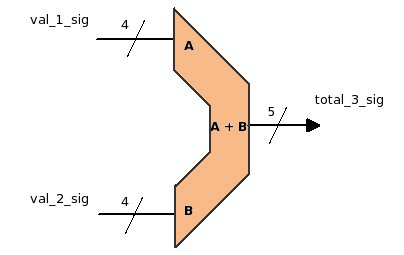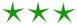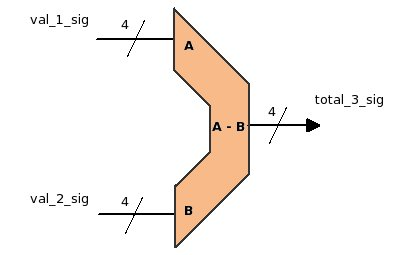black nike shoes roshe cheapnicesports.com

Coding adder or subtracter is not a big deal in VHDL, but what gets implemented in hardware is more important. Given below is a simple adder, subtracter and its respective coding.VHDL Code

---- Signal Declaration ---

```SIGNAL val_1_sig   : STD_LOGIC_VECTOR(3 DOWNTO 0);
SIGNAL val_2_sig   : STD_LOGIC_VECTOR(3 DOWNTO 0);
SIGNAL total_3_sig : STD_LOGIC_VECTOR(3 DOWNTO 0);
```

----- Code region ---

`total_3_sig <= val_1_sig + val_2_sig; `

From the above code you might have noticed that coding adder is just putting a + between two signals, and for this the synthesis tool will implement 4-bit adder. But notice carefully that the input signals (val_1_sig , val_2_sig) are 4 bit wide, but output signal (total_3_sig) is 5 bit wide. This is because the max value of input signal can go upto 15 (decimal) but their addition can reach upto value 30 (decimal). Hence a 5 bit signal for output used instead of4 bits.

Suppose accidentally you used 4 bit signal at output and addition value is more than 15. In this case you will always get the rounded off value (which may not be as desired). In such scenarios neither compiler or the simulator will assert error, so be carefully with width while using adders.

Taking very large width signals (much more than requirement) is also not a good idea. Functionally, above adder will work fine even if the output signal is 10 bits, but in this case if the synthesis tool is unable to optimize the design, then a 10-bit adder will be implemented. This will shoot up the gate usage, since 10 bit adder will require more gates as compare to 4-bit adder.Subtracter

SubtracterVHDL Code

---- Signal Declaration ---

```SIGNAL val_1_sig   : STD_LOGIC_VECTOR(3 DOWNTO 0);
SIGNAL val_2_sig   : STD_LOGIC_VECTOR(3 DOWNTO 0);
SIGNAL total_3_sig : STD_LOGIC_VECTOR(3 DOWNTO 0);
```

----- Code region ---

`total_3_sig <= val_1_sig - val_2_sig; `

Now in case of subtracter notice that the output signal (total_3_sig) is only 4 bit wide.. Why ? It is because the subtraction result will never coss 4 bit value, and hence we need only 4 bit output signal.

The above subtracter will work fine till B is less then A , but will give overflown value if B becomes greater then A.

China is the capital of everything fake. Fake attitude, fake shoes, fake food, fake people, fake history, fake clothes, fake country